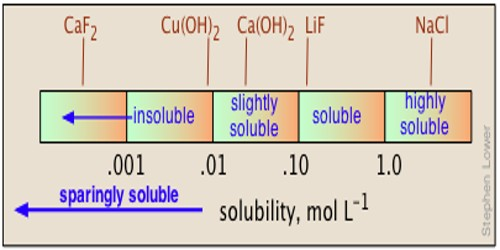Chemistry

# Determination of Solubility of Sparingly Soluble SaltsDetermination of solubility of sparingly soluble salts:

Conductance measurement can be used for measurements of solubility of slightly soluble substances like BaSO4, PbSO4, AgCl etc. The method is based on the difference in the conductance of the ions of the salts. When a sparingly soluble salt is placed in water, a very little amount of the salt dissolves in the water and a solution is created. For the purpose, a saturated solution of the salt is prepared in conductivity water and allowed to stand until almost all the insoluble fraction settles down. The solubility product, KSP, determines the equilibrium that exists between the sparingly soluble salt and the ions that are dissolved in water. The clear solution from the top is taken and its conductance is measured in a conductance cell. The cell is thoroughly washed and filled with the same conductivity water and the conductance of the water measured in the same cell. The observed conductance is then converted to specific conductance. The conductance of water is then subtracted from the conductance of the solution. The value so obtained is the conductance due to the salt.

The solubility of such salts can be calculated by the application of Kholrausch’s law and conductance measurements. The molar conductance (Λm) of a saturated solution as given by equation-

Λm = (κ x 1000)/c

where κ is the specific conductance and ‘c’ is the concentration of the solution in mol L-1. ‘c’ is the concentration of the salt in eq/l and hence the solubility of the salt. Since the salt is sparingly soluble, the solution is very dilute and Λm may be considered to be equal to Λ0m i.e., the molar conductance at infinite dilution. The value of Λ0m tor AgCl can be found by applying Kohlrausch’s law of independent ionic migration. According to this law, Λ0m(AgCl) = λ0ag + λ0Cl. Substituting Λ0m in the above equation ‘c’ can be calculated provided κ is known. This will give the value of ‘c’ in mol L-1 from which the solubility in gL-1 may be calculated. Since the salt dissolves very little, the solutions are very dilute, and since the salt are strong electrolytes.

Conductance offers a very easy and suitable mean for determining the solubility of a sparingly soluble salt such as AgCl, BaSO4, PbSO4, etc. dissolved in H2O to a very small level. That is why these salts are called sparingly soluble salts.